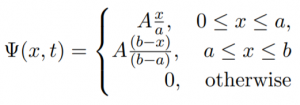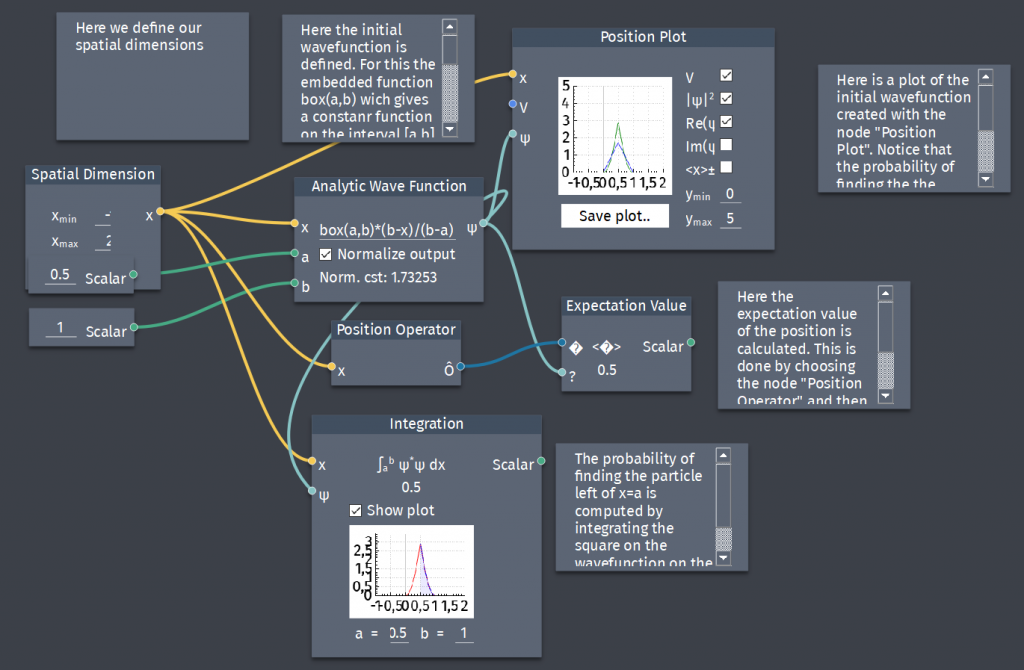# Particle with given wavefunction

At the time $t=0$ a particle is represented by the wave function:where $A,a$ and $b$ are constants.

a) Normalize $\Psi$.
b) Plot $\Psi(x,0)$.
c) Where is the particle most likely to be found at $t=0$?
d) What is the probability of finding the particle left of $x=a$?
e) What is the expectation value of $x$?